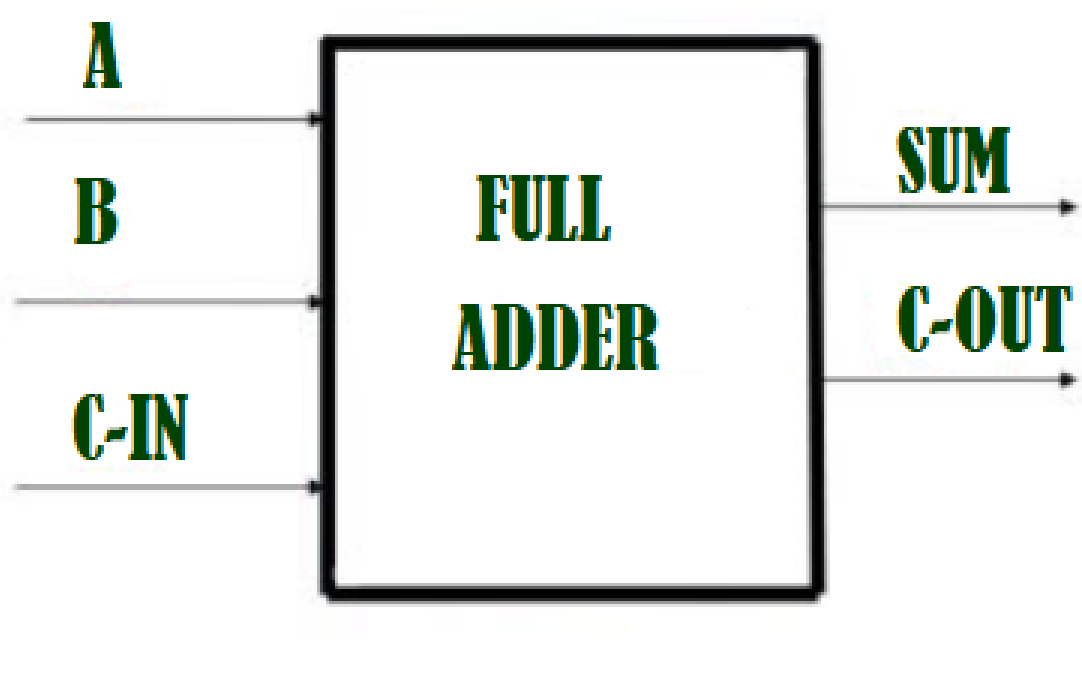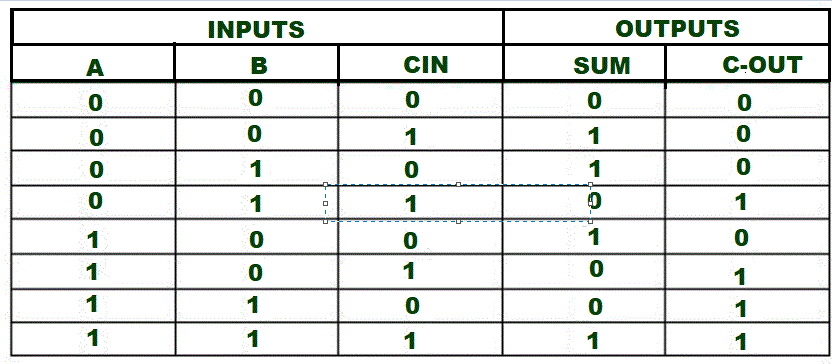# C++ program to implement Full Adder

• Difficulty Level : Expert
• Last Updated : 19 Aug, 2021

We are given three inputs of Full Adder A, B,C-IN. The task is to implement the Full Adder circuit and Print output i.e. sum and C-Out of three inputs.

Introduction :
A Full Adder is a combinational circuit that performs an addition operation on three 1-bit binary numbers. The Full Adder has three input states and two output states. The two outputs are Sum and Carry.

Attention reader! Don’t stop learning now. Get hold of all the important CS Theory concepts for SDE interviews with the CS Theory Course at a student-friendly price and become industry ready.Here we have three inputs A, B, Cin and two outputs Sum, Cout. And the truth table for Full Adder isLogical Expression :

SUM  =  C-IN  XOR  ( A XOR B )
C-0UT= A B + B C-IN + A C-IN

Examples –

1.        Input :   A=1, B=0,C-In=0
Output : Sum=1, C-Out=0
Explanation –
Here from logical expression Sum= C-IN XOR (A XOR B )  i.e. 0 XOR (1 XOR 0) =1 , C-Out= A B + B C-IN + A C-IN              i.e.,  1 AND 0 + 0 AND 0 + 1 AND 0 = 0 .

2.      Input :    A=1, B=1,C-In=0
Output:  Sum=0, C-Out=1

Approach :

• Initialize the variables Sum and C_Out for storing outputs.
• First we will take three inputs A ,B and C_In.
• By applying  C-IN XOR (A XOR B ) we get the value of Sum.
• By applying   A B + B C-IN + A C-IN we get the value of C_Out.

## C++

 `// C++ program to implement full adder``#include ``using` `namespace` `std;``// Function to print sum and C-Out``void` `Full_Adder(``int` `A,``int` `B,``int` `C_In){``    ``int` `Sum , C_Out;``    ``// Calculating value of sum``    ``Sum = C_In ^ (A ^ B);``   ` `    ``//Calculating value of C-Out``    ``C_Out = (A & B) || (B & C_In) || (A & C_In);``  ` `   ``// printing the values``    ``cout<<``"Sum = "``<
Output
```Sum = 1
C-Out = 0```
My Personal Notes arrow_drop_up